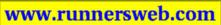## Track Lane Distance Calculator

 Lane Number Lane Width Lane Distance M M Lane width is a minimum of 1.22 metres and a maximum of 1.25 metres

Background:

The formula used in the javascript calculator is:

L = 2S + 2pi(R + (n-1)w) where

L = lane distance,
S = length of the straight
R is the radius of the turn
n is the lane number
w is the width of the lane.

Therefore the extra distance run in lane n for 1 lap is: 2S + 2pi(R + (n - 1)r) - 2S - 2piR which = 2pi(n-1)r

The total distance run is (N(400 + 2pi(n - 1)r)

The JavaScript formula is: LaneDistance = (400) + (( 2 * 22/7) * (track_Lane - 1) * track_Lane_Width).

When is a mile not a mile from Peak Performance Online.